Mathematics: CUET Mock Test - 1

# Mathematics: CUET Mock Test - 1

Test Description

## 40 Questions MCQ Test CUET Science Subjects Mock Tests | Mathematics: CUET Mock Test - 1

Mathematics: CUET Mock Test - 1 for Class 12 2023 is part of CUET Science Subjects Mock Tests preparation. The Mathematics: CUET Mock Test - 1 questions and answers have been prepared according to the Class 12 exam syllabus.The Mathematics: CUET Mock Test - 1 MCQs are made for Class 12 2023 Exam. Find important definitions, questions, notes, meanings, examples, exercises, MCQs and online tests for Mathematics: CUET Mock Test - 1 below.
Solutions of Mathematics: CUET Mock Test - 1 questions in English are available as part of our CUET Science Subjects Mock Tests for Class 12 & Mathematics: CUET Mock Test - 1 solutions in Hindi for CUET Science Subjects Mock Tests course. Download more important topics, notes, lectures and mock test series for Class 12 Exam by signing up for free. Attempt Mathematics: CUET Mock Test - 1 | 40 questions in 45 minutes | Mock test for Class 12 preparation | Free important questions MCQ to study CUET Science Subjects Mock Tests for Class 12 Exam | Download free PDF with solutions
 1 Crore+ students have signed up on EduRev. Have you?
Mathematics: CUET Mock Test - 1 - Question 1

### Which of these is not a type of relation?

Detailed Solution for Mathematics: CUET Mock Test - 1 - Question 1

Surjective is not a type of relation. It is a type of function. Reflexive, Symmetric and Transitive are type of relations.

Mathematics: CUET Mock Test - 1 - Question 2

### Let a binary operation ‘*’ be defined on a set A. The operation will be commutative if ________

Detailed Solution for Mathematics: CUET Mock Test - 1 - Question 2

A binary operation ‘*’ defined on a set A is said to be commutative only if a * b = b *a, ∀ a, b ∈ A.
If (a * b) * c = a * (b * c), then the operation is said to associative ∀ a, b∈ A.
If (b ο c) * a = (b * a) ο (c * a), then the operation is said to be distributive ∀ a, b, c ∈ A.

Mathematics: CUET Mock Test - 1 - Question 3

### tan−1√3+sec−12–cos−11 is equal to ________

Detailed Solution for Mathematics: CUET Mock Test - 1 - Question 3

tan−1 √3 = π/3, sec−12 = π/3, cos−11 = 0
tan−1√3 + sec−12 – cos−11 = π/3 + π/3
= 2π/3

Mathematics: CUET Mock Test - 1 - Question 4

sin-1⁡x in terms of cos-1⁡ is _________

Detailed Solution for Mathematics: CUET Mock Test - 1 - Question 4

Let sin-1⁡x = y
⇒ x = sin⁡y
⇒ x = √1 - cos2y
⇒ x2 = 1 - cos2y
⇒ cos2y = 1 - x2
∴ y = cos-1⁡ √1 - x2 = sin-1⁡x

Mathematics: CUET Mock Test - 1 - Question 5

Which of the following relations is symmetric but neither reflexive nor transitive for a set A = {1, 2, 3}.

Detailed Solution for Mathematics: CUET Mock Test - 1 - Question 5

A relation in a set A is said to be symmetric if (a1, a2)∈R implies that (a1, a2)∈R,for every a1, a2∈R.
Hence, for the given set A={1, 2, 3}, R={(1, 2), (2, 1)} is symmetric. It is not reflexive since every element is not related to itself and neither transitive as it does not satisfy the condition that for a given relation R in a set A if (a1, a2)∈R and (a2, a3)∈R implies that (a1, a3)∈ R for every a1, a2, a3∈R.

Mathematics: CUET Mock Test - 1 - Question 6

If f : R→R, g(x) = 3 x 2 + 7 and f(x) = √x, then gοf(x) is equal to _______

Detailed Solution for Mathematics: CUET Mock Test - 1 - Question 6

Given that, g(x) = 3 x 2 + 7 and f(x) = √x
∴ gοf(x) = g(f(x)) = g(√x) = 3(√x)2 + 7 = 3x + 7.
Hence, gοf(x) = 3x + 7.

Mathematics: CUET Mock Test - 1 - Question 7

Let I be a set of all lines in a XY plane and R be a relation in I defined as R = {(I1, I2):I1 is parallel to I2}. What is the type of given relation?

Detailed Solution for Mathematics: CUET Mock Test - 1 - Question 7

This is an equivalence relation. A relation R is said to be an equivalence relation when it is reflexive, transitive and symmetric.
Reflexive: We know that a line is always parallel to itself. This implies that I1 is parallel to I1 i.e. (I1, I2)∈R. Hence, it is a reflexive relation.
Symmetric: Now if a line I1 || I2 then the line I2 || I1. Therefore, (I1, I2)∈R implies that (I2, I1)∈R. Hence, it is a symmetric relation.
Transitive: If two lines (I1, I3) are parallel to a third line (I2) then they will be parallel to each other i.e. if (I1, I2) ∈R and (I2, I3) ∈R implies that (I1, I3) ∈R.

Mathematics: CUET Mock Test - 1 - Question 8

What is the principle value of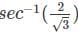.

Detailed Solution for Mathematics: CUET Mock Test - 1 - Question 8

Let= y
sec y = 2/√3
sec⁡ y = secπ/6
⇒ y = π/6

Mathematics: CUET Mock Test - 1 - Question 9

What is sec-1⁡x in terms of tan-1⁡?

Detailed Solution for Mathematics: CUET Mock Test - 1 - Question 9

Let sec-1⁡x = y
⇒ x = sec⁡y
⇒ x = √ 1 + tan2y
⇒ x2 - 1 = tan2y
∴ y = tan-1√x2 - 1 = sec-1⁡x

Mathematics: CUET Mock Test - 1 - Question 10

If A =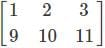and B =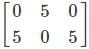, then find A + B.

Detailed Solution for Mathematics: CUET Mock Test - 1 - Question 10

Given that, A =and B =Then A + B =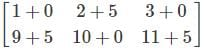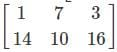Mathematics: CUET Mock Test - 1 - Question 11

If f : R → R is given by f(x) = (5 + x4)1/4, then fοf(x) is _______

Detailed Solution for Mathematics: CUET Mock Test - 1 - Question 11

Given that f(x) = (5 + x4)1/4
∴ fοf(x) = f(f(x)) = (5 + {(5 + x4)1/4}4)1/4
= (5 + (5 + x4))1/4 = (10+x4)1/4

Mathematics: CUET Mock Test - 1 - Question 12

Let ‘*’ be a binary operation on N defined by a * b =a - b + ab2, then find 4 * 5.

Detailed Solution for Mathematics: CUET Mock Test - 1 - Question 12

The binary operation is defined by a * b = a - b + ab2.
∴ 4 * 5 = 4 - 5 + 4(52) = -1 + 100 = 99.

Mathematics: CUET Mock Test - 1 - Question 13

[-1, 1] is the domain for which of the following inverse trigonometric functions?

Detailed Solution for Mathematics: CUET Mock Test - 1 - Question 13

[-1, 1] is the domain for sin-1⁡x.
The domain for cot-1⁡x is (-∞,∞).
The domain for tan-1⁡⁡x is (-∞,∞).
The domain for sec-1⁡⁡x is (-∞,-1] ∪ [1,∞).

Mathematics: CUET Mock Test - 1 - Question 14

If A + B =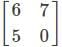and A =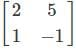. Find the matrix B.

Detailed Solution for Mathematics: CUET Mock Test - 1 - Question 14

Given that,
A + B =and A =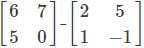⇒ B = (A + B) - A =B =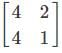Mathematics: CUET Mock Test - 1 - Question 15

A function is invertible if it is ____________

Detailed Solution for Mathematics: CUET Mock Test - 1 - Question 15

A function is invertible if and only if it is bijective i.e. the function is both injective and surjective. If a function f: A → B is bijective, then there exists a function g: B → A such that f(x) = y ⇔ g(y) = x, then g is called the inverse of the function.

Mathematics: CUET Mock Test - 1 - Question 16

Let M={7,8,9}. Determine which of the following functions is invertible for f:M→M.

Detailed Solution for Mathematics: CUET Mock Test - 1 - Question 16

The function f = {(7,7),(8,8),(9,9)} is invertible as it is both one – one and onto. The function is one – one as every element in the domain has a distinct image in the co – domain. The function is onto because every element in the codomain M = {7,8,9} has a pre – image in the domain.

Mathematics: CUET Mock Test - 1 - Question 17

Let R be a relation in the set N given by R={(a,b): a+b=5, b>1}. Which of the following will satisfy the given relation?

Detailed Solution for Mathematics: CUET Mock Test - 1 - Question 17

(2,3) ∈ R as 2+3 = 5, 3>1, thus satisfying the given condition.
(4,2) doesn’t belong to R as 4+2 ≠ 5.
(2,1) doesn’t belong to R as 2+1 ≠ 5.
(5,0) doesn’tbelong to R as 0⊁1

Mathematics: CUET Mock Test - 1 - Question 18

If f: N→N, g: N→N and h: N→R is defined f(x) = 3x - 5, g(y) = 6y2 and h(z) = tan⁡z, find ho(gof).

Detailed Solution for Mathematics: CUET Mock Test - 1 - Question 18

Given that, f(x) = 3x - 5, g(y) = 6y2 and h(z) = tan⁡z,
Then, ho(gof) = hο(g(f(x)) = h(6(3x-5)2) = tan⁡(6(3x - 5)2)
∴ ho(gof) = tan⁡(6(3x - 5)2)

Mathematics: CUET Mock Test - 1 - Question 19

Let ‘*’ be defined on the set N. Which of the following are both commutative and associative?

Detailed Solution for Mathematics: CUET Mock Test - 1 - Question 19

The binary operation ‘*’ is both commutative and associative for a * b = a + b.
The operation is commutative on a * b = a + b because a + b = b + a.
The operation is associative on a * b = a + b because (a + b) + c = a + (b + c).

Mathematics: CUET Mock Test - 1 - Question 20

Let ‘*’ be a binary operation defined by a * b = 4ab. Find (a * b) * a.

Detailed Solution for Mathematics: CUET Mock Test - 1 - Question 20

Given that, a * b = 4ab.
Then, (a * b) * a = (4ab) * a
= 4(4ab)(a) = 16a2 b.

Mathematics: CUET Mock Test - 1 - Question 21

(a,a) ∈ R, for every a ∈ A. This condition is for which of the following relations?

Detailed Solution for Mathematics: CUET Mock Test - 1 - Question 21

The above is the condition for a reflexive relation. A relation is said to be reflexive if every element in the set is related to itself.

Mathematics: CUET Mock Test - 1 - Question 22

Let ‘*’ be a binary operation defined by a * b = 3ab + 5. Find 8 * 3.

Detailed Solution for Mathematics: CUET Mock Test - 1 - Question 22

It is given that a * b=3ab + 5.
Then, 8 * 3 = 3(83) + 5 = 3(512) + 5 = 1536 + 5 = 1541.

Mathematics: CUET Mock Test - 1 - Question 23

Which of the following is not a type of binary operation?

Detailed Solution for Mathematics: CUET Mock Test - 1 - Question 23

Transitive is not a type of binary operation. It is a type of relation. Distributive, associative, commutative are different types of binary operations.

Mathematics: CUET Mock Test - 1 - Question 24

Which of the following relations is reflexive but not transitive for the set T = {7, 8, 9}?

Detailed Solution for Mathematics: CUET Mock Test - 1 - Question 24

The relation R= {(7, 7), (8, 8), (9, 9)} is reflexive as every element is related to itself i.e. (a,a) ∈ R, for every a∈A. and it is not transitive as it does not satisfy the condition that for a relation R in a set A if (a1, a2)∈R and (a2, a3)∈R implies that (a1, a3) ∈ R for every a1, a2, a3 ∈ R.

Mathematics: CUET Mock Test - 1 - Question 25

Which of the following condition is incorrect for matrix multiplication?

Detailed Solution for Mathematics: CUET Mock Test - 1 - Question 25

Matrix multiplication is never commutative i.e. AB ≠ BA. Therefore, the condition AB = BA is incorrect.

Mathematics: CUET Mock Test - 1 - Question 26

Which of the following is not the property of transpose of a matrix?

Detailed Solution for Mathematics: CUET Mock Test - 1 - Question 26

(AB)’ = (BA)’is incorrect. We know that matrix multiplication is not commutative i.e. AB ≠ BA. Hence, its transpose will also not be commutative.
(AB)’=B’A’

Mathematics: CUET Mock Test - 1 - Question 27

Find the transpose of the matrix A =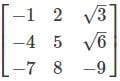Detailed Solution for Mathematics: CUET Mock Test - 1 - Question 27

To find the transpose of the matrix of the given matrix, interchange the rows with columns and columns with rows.
Hence, we get A’ =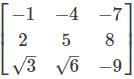Mathematics: CUET Mock Test - 1 - Question 28

Which of the following is the reversal law of transposes?

Detailed Solution for Mathematics: CUET Mock Test - 1 - Question 28

According to the reverse law of transposes the transpose of the product is the product of the transposes taken in the reverse order i.e. (AB)’ = B’ A’.

Mathematics: CUET Mock Test - 1 - Question 29

The matrix A =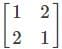is a ____________

Detailed Solution for Mathematics: CUET Mock Test - 1 - Question 29

Given that, A =⇒ A’ =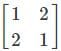i.e.A=A’. Hence, it is a symmetric matrix.

Mathematics: CUET Mock Test - 1 - Question 30

Which of the following conditions holds true for a skew-symmetric matrix?

Detailed Solution for Mathematics: CUET Mock Test - 1 - Question 30

A matrix is said to be skew-symmetric if it is equal to the negative of its transpose i.e. A = -A’.

Mathematics: CUET Mock Test - 1 - Question 31

The matrix A =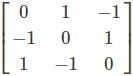is __________

Detailed Solution for Mathematics: CUET Mock Test - 1 - Question 31

The given matrix A =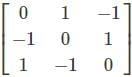is skew symmetric.
⇒ A’ =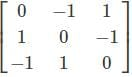= A
∴ A = -A’. Hence, it is a skew-symmetric matrix.

Mathematics: CUET Mock Test - 1 - Question 32

If A =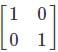, then which of the following statement is incorrect?

Detailed Solution for Mathematics: CUET Mock Test - 1 - Question 32

Given that, A =∴ A’ =⇒ -A ’=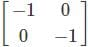≠ A. Hence, it is not a skew symmetric matrix.

Mathematics: CUET Mock Test - 1 - Question 33

Which of the following conditions holds true for a symmetric matrix?

Detailed Solution for Mathematics: CUET Mock Test - 1 - Question 33

A matrix is A said to be a symmetric matrix if it is equal to its transpose i.e. A = A’.

Mathematics: CUET Mock Test - 1 - Question 34

How many elementary operations are possible on Matrices?

Detailed Solution for Mathematics: CUET Mock Test - 1 - Question 34

There are a total of 6 elementary operations that are possible on matrices, three on rows and three on columns.

Mathematics: CUET Mock Test - 1 - Question 35

Which of the following matrices will remain same if the elementary operation R1 → 2R1 + 3R2 is applied on the matrix?

Detailed Solution for Mathematics: CUET Mock Test - 1 - Question 35

Consider matrix A =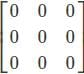, applying the elementary operation R1 → 2R1 + 3R2.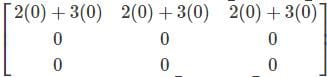herefore, the matrix A =, remains same after applying the elementary operation.

Mathematics: CUET Mock Test - 1 - Question 36

Which of the following is not a valid elementary operation?

Detailed Solution for Mathematics: CUET Mock Test - 1 - Question 36

The elementary operation Ri →1 + kRi is incorrect, the valid elementary operations on matrices are as follows.

• Interchanging any two rows and columns
• The multiplication of the elements of any row or column by a non-zero number.
• The addition to the elements of any row or column, the corresponding elements of any other row and column multiplied by any non-zero number.
Mathematics: CUET Mock Test - 1 - Question 37

Which of the following column operation is incorrect for the matrix A =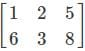?

Detailed Solution for Mathematics: CUET Mock Test - 1 - Question 37

The column operation C2→2+2C2 is incorrect. A non-zero number cannot be directly added to any column or row in a matrix.

Mathematics: CUET Mock Test - 1 - Question 38

Which of the following relations is transitive but not reflexive for the set S = {3, 4, 6}?

Detailed Solution for Mathematics: CUET Mock Test - 1 - Question 38

For the above given set S = {3, 4, 6}, R = {(3, 4), (4, 6), (3, 6)} is transitive as (3,4)∈R and (4,6) ∈R and (3,6) also belongs to R . It is not a reflexive relation as it does not satisfy the condition (a,a)∈R, for every a∈A for a relation R in the set A.

Mathematics: CUET Mock Test - 1 - Question 39

Which of the following relations is symmetric and transitive but not reflexive for the set I = {4, 5}?

Detailed Solution for Mathematics: CUET Mock Test - 1 - Question 39

R= {(4, 5), (5, 4), (4, 4)} is symmetric since (4, 5) and (5, 4) are converse of each other thus satisfying the condition for a symmetric relation and it is transitive as (4, 5)∈R and (5, 4)∈R implies that (4, 4) ∈R. It is not reflexive as every element in the set I is not related to itself.

Mathematics: CUET Mock Test - 1 - Question 40

Which of the following is not a property of invertible matrices if A and B are matrices of the same order?

Detailed Solution for Mathematics: CUET Mock Test - 1 - Question 40

(AB)-1 = A-1 B-1 is incorrect. The correct formula is (AB)-1 = B-1 A-1. B-1 A-1 ≠  A-1 B-1 as matrix multiplication is not commutative.

## CUET Science Subjects Mock Tests

60 tests
 Use Code STAYHOME200 and get INR 200 additional OFF Use Coupon Code
Information about Mathematics: CUET Mock Test - 1 Page
In this test you can find the Exam questions for Mathematics: CUET Mock Test - 1 solved & explained in the simplest way possible. Besides giving Questions and answers for Mathematics: CUET Mock Test - 1, EduRev gives you an ample number of Online tests for practice

60 tests